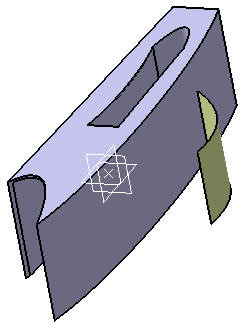# Transform AffinityThe Transform feature is a type of feature modifier. This capability modifies a set of functional features of the active body by applying a transformation to them individually. The affected features retain their full functional behavior.

• The command allows you to define four types of transformations:
•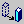Translation - Translates one or more features.
•Rotation - Rotates one or more features about an axis.
•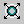Scaling - Resizes one or more features based on a ratio factor.
•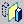Symmetry - Applies a Symmetry transformation by reflection.
•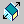Affinity - Transforms the absolute size by multiplying a scale ratio.
•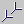Axis to Axis - Transposes the geometry from one reference axis system to another.

An Affinity transformation changes the absolute size of the selected functional features by multiplying a scale ratio in a given direction to a geometric coordinate value. Unlike the Scale transformation, each direction of the referenced axis system may use a different scale factor. If the scaling ratios for the three directions are dissimilar, you may result in a deformed features.

The following inputs are required:

Axis system: By specifying an origin, the XY plane, and the direction of the X axis, the system will create a reference axis system that the scaling ratios will be applied to.

• Origin: The new reference axis origin.
• XY plane: The XY plane of the new reference axis system.
• X axis: The X axis of the new reference axis system.

Ratios: Specifies the scaling factor in a particular axis direction. The user may specify a scaling factor for the X, Y, and Z axes. Default is “1.0” for each direction.

• X Ratio: the scale to apply to the x axis.
• Y Ratio: the scale to apply to the y axis
• Z Ratio: the scale to apply to the z axis.

This scenario illustrates an Affinity transform applied to two functional features at the same time (Shellable Prism and Pocket).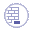Open the Transform.CATPart document.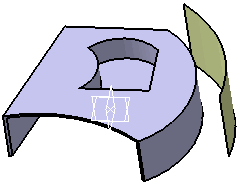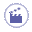1. Click the Transform icon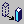. The Transform dialog box is displayed.

Set Transform type:  Affinity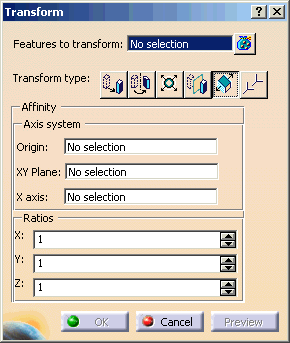2. Select Features to transform: Shellable Prism.1 and Pocket.1.

3. Select Origin: Select Point.1

4. Select XY Plane: Select xy plane

5. Select X axis: Select X Axis using RMB

6.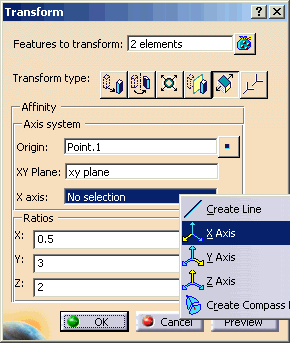7. Enter X=0.5, Y=3, Z=2 for Ratios.

8. Click OK.

9.